Question

# Write the complete decay equation for the given nuclide in the complete A z XN notation....

Write the complete decay equation for the given nuclide in the complete

 A z

XN notation. Refer to the periodic table for values of Z.

(a)

Write the complete α decay equation for 247Bk.

(b)

Find the energy released (in MeV) in the decay. (Assume 1 u = 931.5 MeV/c2.)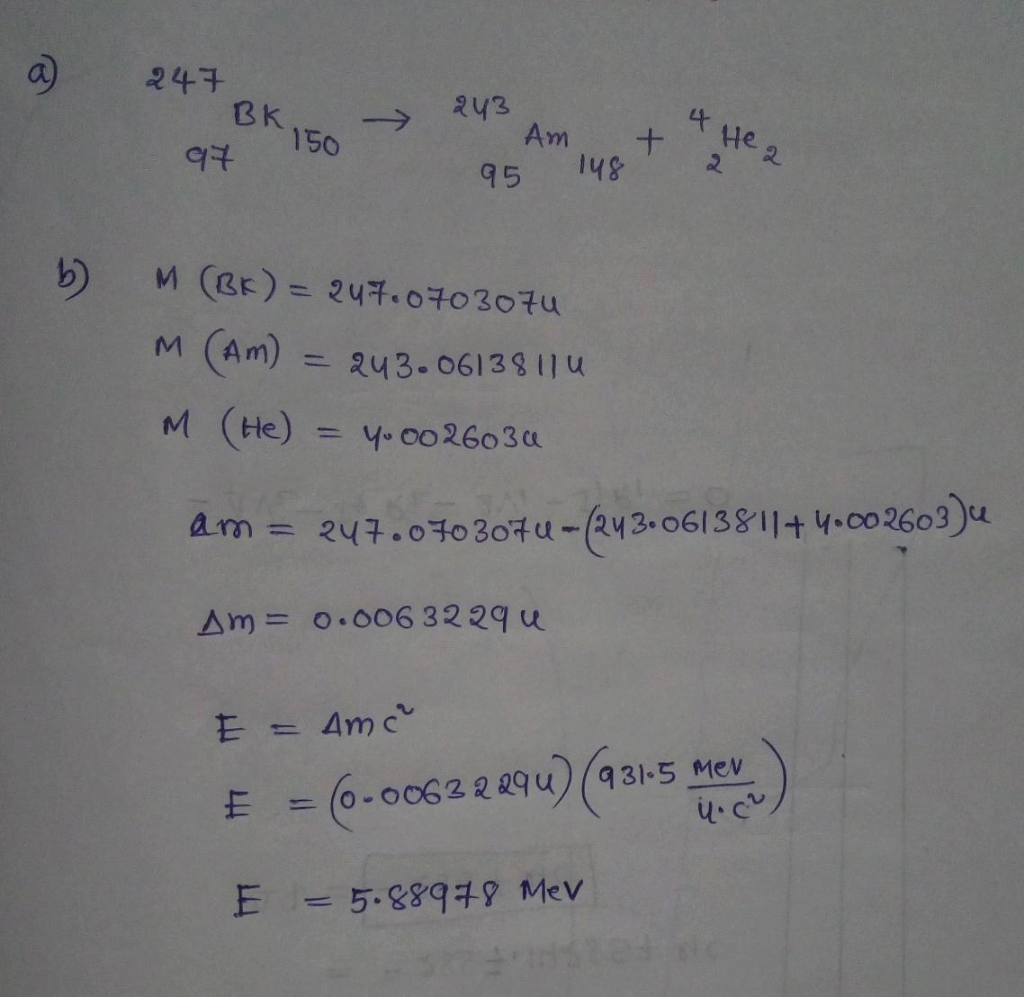#### Earn Coins

Coins can be redeemed for fabulous gifts.

Similar Homework Help Questions
• ### Write the complete decay equation for the given nuclide in the complete y notation. Refer to...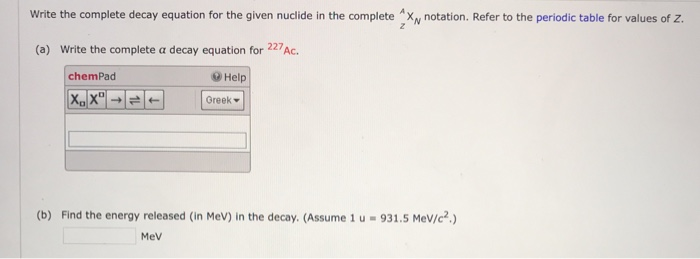Write the complete decay equation for the given nuclide in the complete y notation. Refer to the periodic table for values of z. (a) Write the complete a decay equation for 227AC. chemPad Help X.," Greek (b) Find the energy released in Mev) in the decay. (Assume 1 u = 931.5 MeV/c?) MeV

• ### Write the complete decay equation for the given nuclide in the complete "X, notation. Refer to...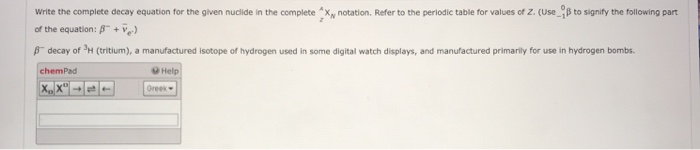Write the complete decay equation for the given nuclide in the complete "X, notation. Refer to the periodic table for values of Z. (Use_B to signify the following part of the equation: 8" +1) f decay of PH (tritium), a manufactured isotope of hydrogen used in some digital watch displays, and manufactured primarily for use in hydrogen bombs. chemPad Help Greek

• ### (a) Write the decay equation for the a decay of 228 *Th. (Enter your answer in...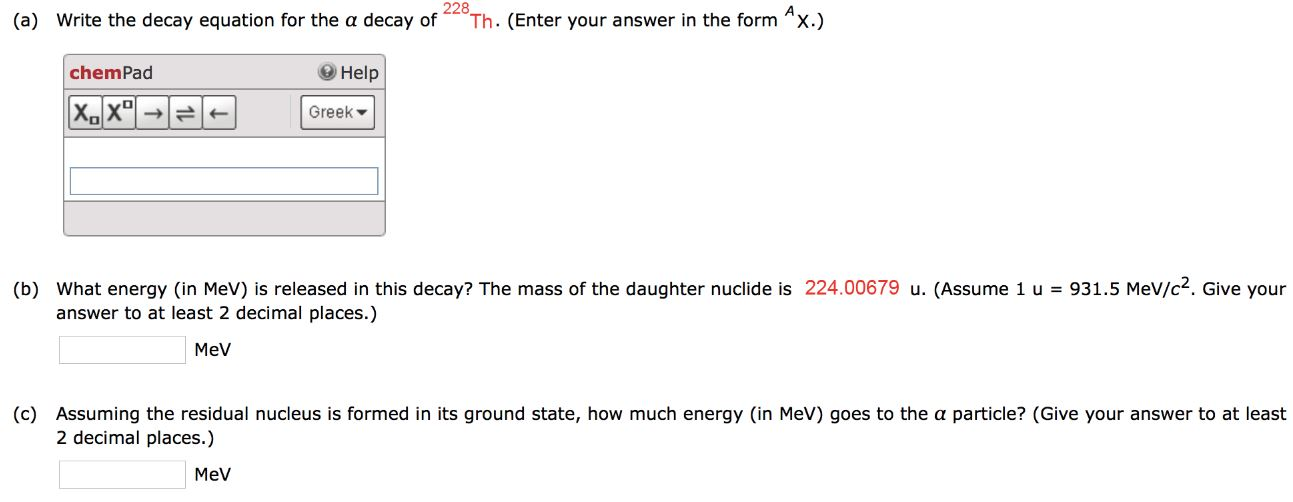(a) Write the decay equation for the a decay of 228 *Th. (Enter your answer in the form ^x.) chemPad Help x. Greek (b) What energy (in MeV) is released in this decay? The mass of the daughter nuclide is 224.00679 u. (Assume 1 u = 931.5 MeV/c2. Give your answer to at least 2 decimal places.) MeV (c) Assuming the residual nucleus is formed in its ground state, how much energy (in MeV) goes to the a particle? (Give...

• ### (a)Calculate the energy (in MeV) released in the α decay of 236U. (Assume 1 u =...

(a)Calculate the energy (in MeV) released in the α decay of 236U. (Assume 1 u = 931.5 MeV/c2.) (b)What fraction of the mass of a single 236U is destroyed in the decay? The mass of 232Th is 232.038054 u.

• ### I am confused and need to see steps to understand (a) Write the complete reaction equation for electron capture by...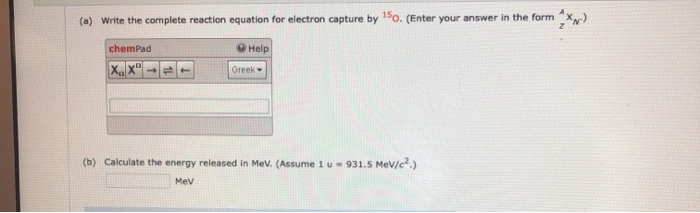I am confused and need to see steps to understand (a) Write the complete reaction equation for electron capture by "So. (Enter your answer in the form Xx) chemPad Help Greek (b) Calculate the energy released in MeV. (Assume 1 u-931.5 MeV/c.) Mev

• ### Nuclear Physics - Alpha Decay a - decay After Before a Parent Daughter 4P→ D +...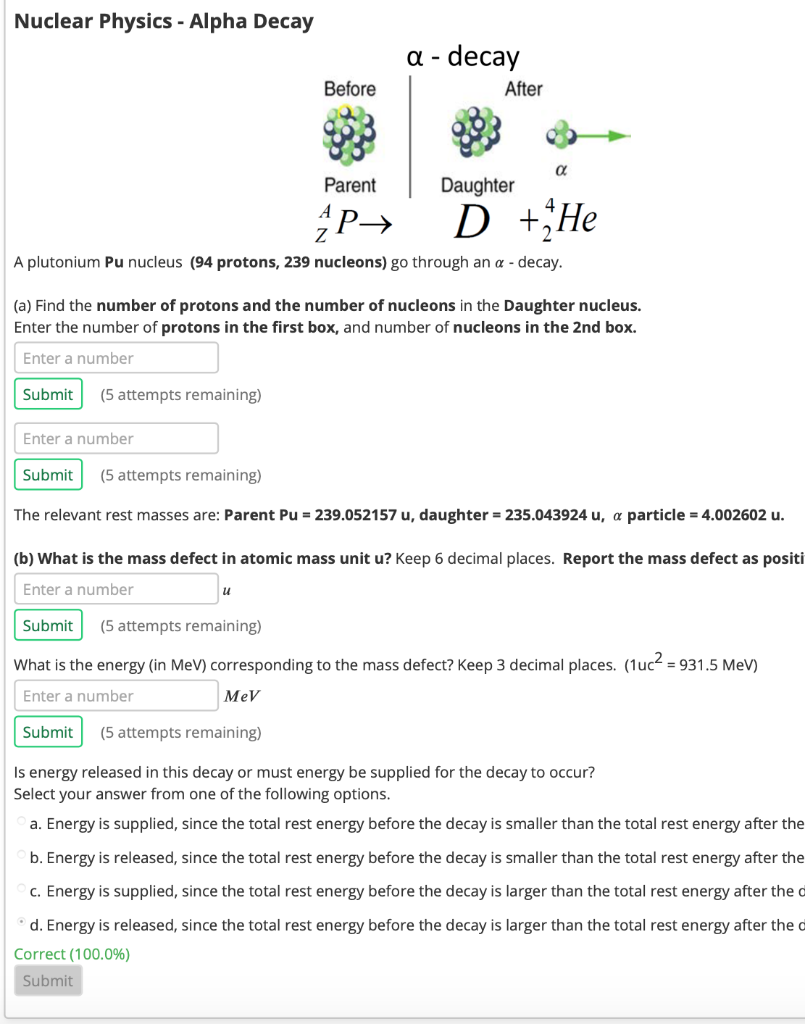Nuclear Physics - Alpha Decay a - decay After Before a Parent Daughter 4P→ D + He Z A plutonium Pu nucleus (94 protons, 239 nucleons) go through an a - decay. (a) Find the number of protons and the number of nucleons in the Daughter nucleus. Enter the number of protons in the first box, and number of nucleons in the 2nd box. Enter a number Submit (5 attempts remaining) Enter a number Submit (5 attempts remaining) The relevant...

• ### answer all questions please (a) Show that, for large Z and A, the energy released when...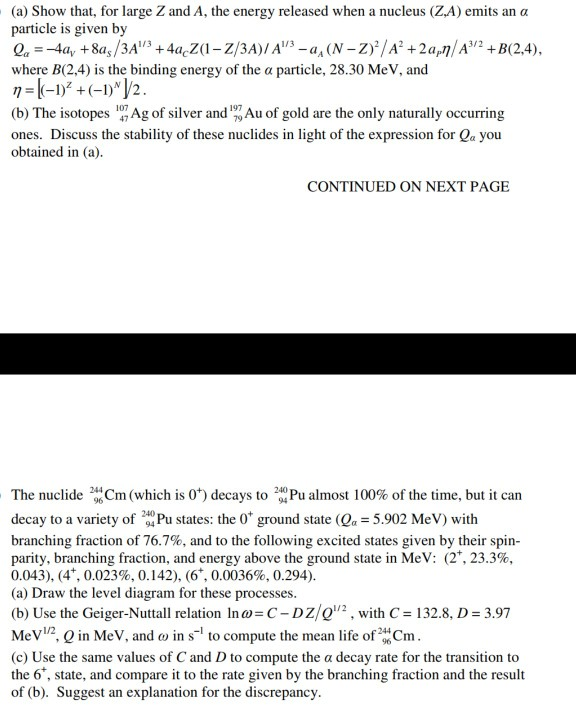answer all questions please (a) Show that, for large Z and A, the energy released when a nucleus (ZA) emits an α particle is given by where B(24) is the binding energy of the α particle, 28.30 MeV, and η =k-1)" + (-1)"/2 (b) The isotopes 17 Ag of silver and Au of gold are the only naturally occurring ones. Discuss the stability of these nuclides in light of the expression for Qa you obtained in (a) CONTINUED ON NEXT...

• ### 8 decay Before B decay Before After After V. Parent Daughter Parent Daughter nen AP →...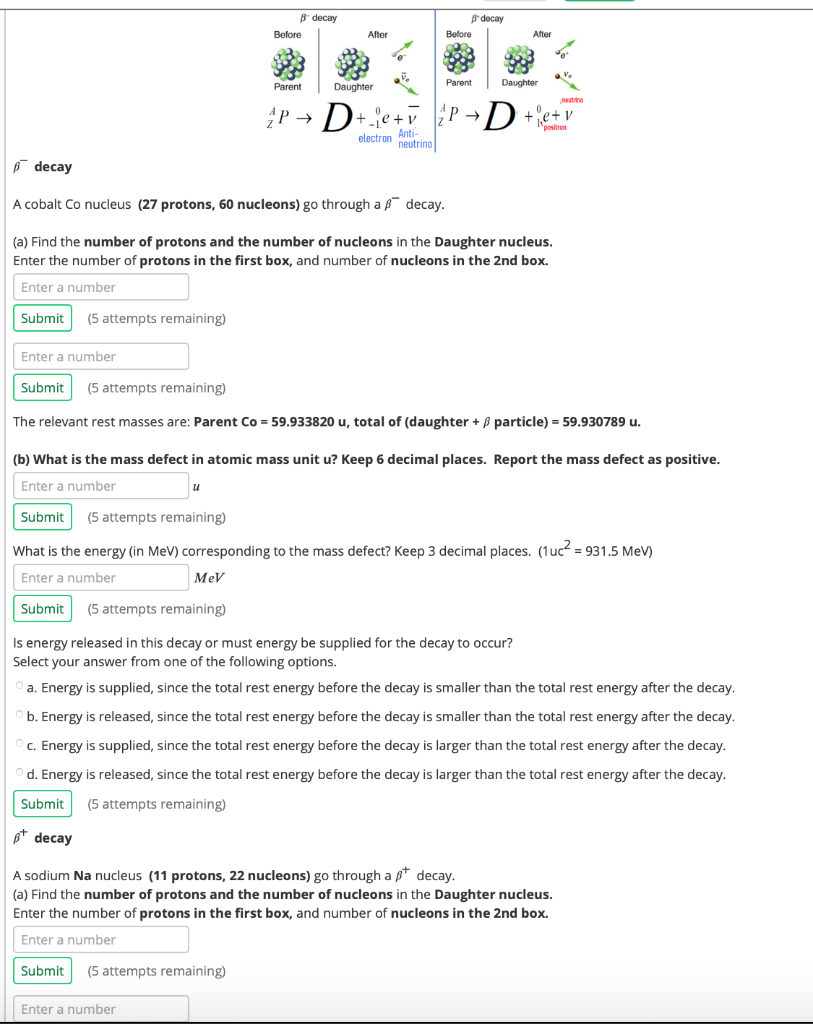8 decay Before B decay Before After After V. Parent Daughter Parent Daughter nen AP → D+e+v P-D + entry electron neutrino Anti- decay A cobalt Co nucleus (27 protons, 60 nucleons) go through a decay. (a) Find the number of protons and the number of nucleons in the Daughter nucleus. Enter the number of protons in the first box, and number of nucleons in the 2nd box. Enter a number Submit (5 attempts remaining) Enter a number Submit (5...

• ### 32. Write a nuclear equation for the indicated decay of each nuclide: a. Po-210 (alpha) b....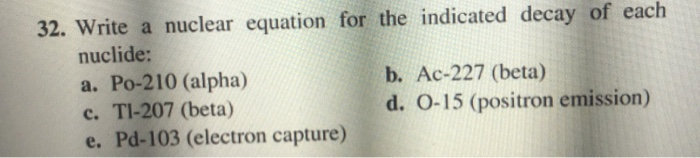32. Write a nuclear equation for the indicated decay of each nuclide: a. Po-210 (alpha) b. Ac-227 (beta) c. T1-207 (beta) d. 0-15 (positron emission) e. Pd-103 (electron capture)

• ### Based on the band of stability, write a balanced equation for one nuclear decay process that each...

Based on the band of stability, write a balanced equation for one nuclear decay process that each of the following nuclides will undergo. (Enter your answer in the form A X Z .) (a) nuclide with 21 protons and 32 neutrons and (b) nuclide with 76 protons and 100 neutrons )